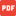# Wawasan Volume I – 2 Benedictus Sonny Yoedono

Aplikasi Metode Beda Hingga (Finite Difference) untuk
Optimasi Tebal Pelat

ABSTRACT

Numerical method has a lot of advantages, furthermore when the research specimen was very small conversely. Numerical method can be used as pre assessment, before laboratory research will to do. The finite difference method is one of numerical method. In this method derivative of differential equation was replaced by difference in the chosen point. Classical plate solution will be applicable if the geometry boundary condition and plate load not too complex. This problem will make the analysis become long and impossible to do. Finite difference method will approach plate solution effectively. This research work through finite difference method application to determine optimum plate thickness with optimum deflection. The specimen was rectangular reinforced concrete plate with 5 m side length, maximum allowable deflection l 360 , poisson ratio ( ) = 0,25, and ‘c f = 30 MPa. Uniform dead load (qD) and life load (qL) applied to the plate. The result are, optimal plate thickness is 115 mm with the optimal defelction 13,455 mm (< allowable defelction = 13,889 mm).

Keywords: finite difference method, plate thickness optimizing, deflection

halaman 49 – 64

File Dokumen dapat di unduh disiniuniversitas katolik widya karya WAWASAN 2013 benedictus.pdf